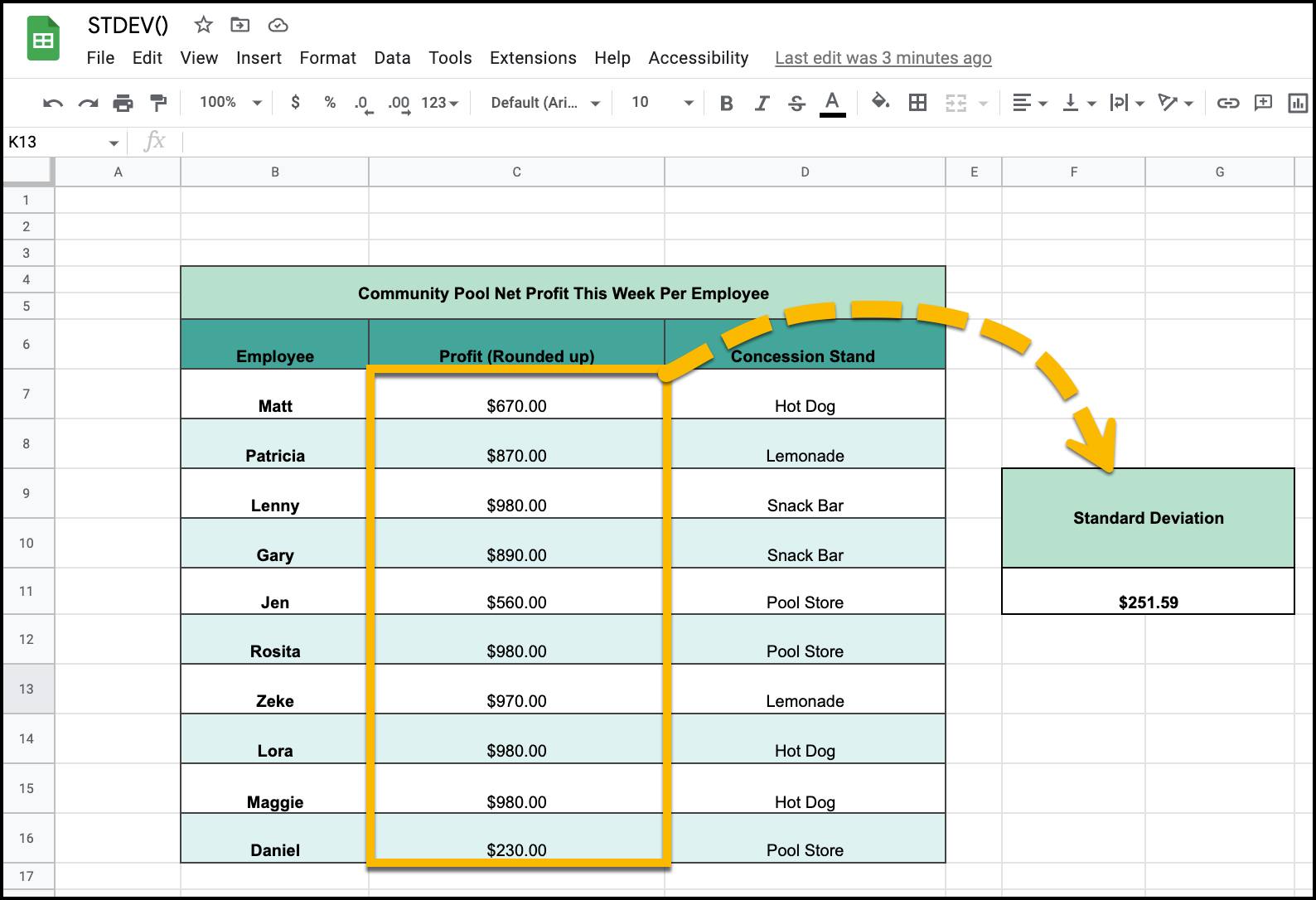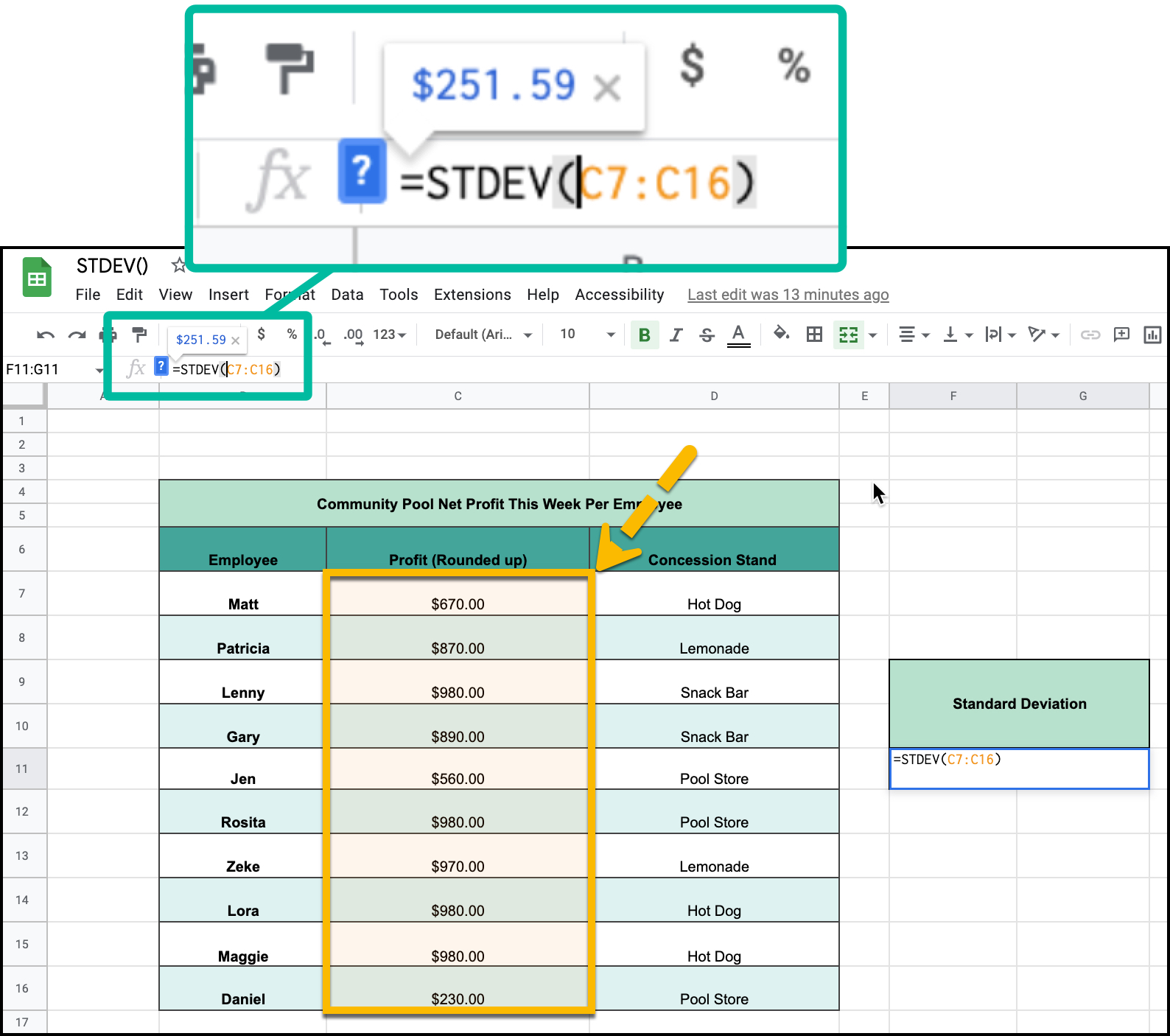# How to Calculate Standard Deviation Using Google Sheets

Standard Deviation is an incredibly powerful data statistic, calculating average variation of data sets. Next to Average, Median, and Sum, it is one of the most popular functions used in google sheets.I will show you how you can use this function in google sheets in this straightforward guide.

## =STDEV(Cell Range)

This is a simple formula. In the function window, enter =STDEV() followed by the cell range of values that you wish to calculate.

In this example I am calculating the imaginary standard deviation of an imaginary set of concession stand employees.And there you have it, that is how you can calculate the standard deviation of any data set in Google Sheets!